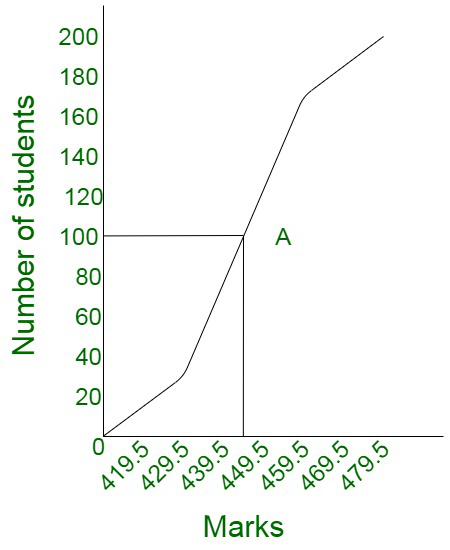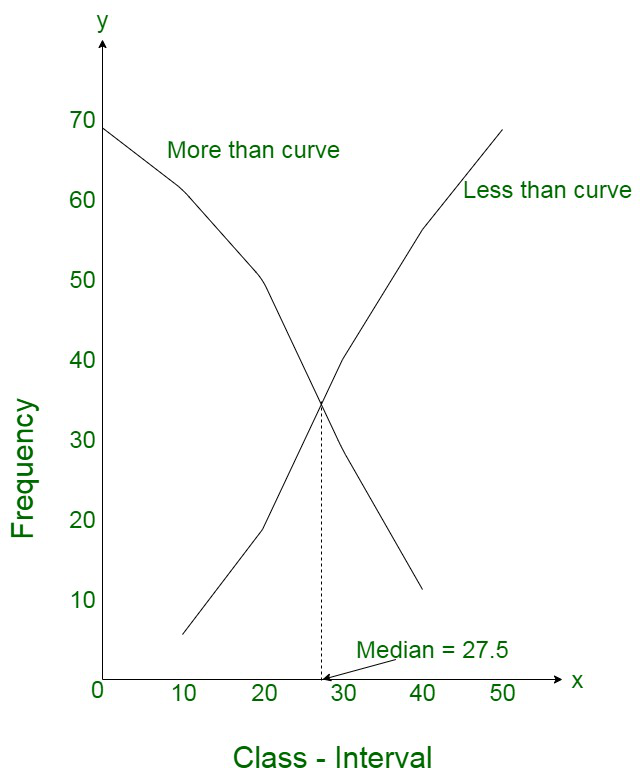Open in App
Not now

# Which measure of central tendency can be determined graphically?

• Last Updated : 13 Oct, 2021

In statistical data, the descriptive summary of a data set is known as the central tendency. It is considered to be an indicator of the center of the data distribution. To summarise, it represents the single value of the entire distribution or a dataset. It describes the data precisely.

However, the central tendency of the data distribution does not provide information regarding individual data from the dataset. There are various measures of the central tendency of the data.

### Measures of Central Tendency

Since we described, the central tendency of the data as an indicator of the values, describing the dataset by computing the central position of the data. The central tendency of the data can be measured by the following quantities:

• Mean: Mean is considered to be the sum of all observations divided by the total number of observations.
• Median: Median is defined as the middle or central value in an ordered set.
• Mode: Mode is defined as the most frequently occurring value in a data set.

The measure of central tendency that can be determined graphically is median

Merits or Uses of Median:

• Median is considered to be rigidly defined like Mean
• Median remains unaffected even in case the value of the extreme item is much different from other values. For instance, the Median in the case of the given numbers, 4, 7, 12, 18, 19 is 12. In case, we add two integral values equivalent to 450 and 10000, the new median obtained is 18.
• Can be used for Quantities.
• Can be located graphically
• In the case of the open-end intervals, calculating the median is the perfect option.
• Easy to understand.
• Easy to calculate.
• Used for statistical devices such as Mean Deviation and skewness.
• Can be calculated by inspection.

For instance, let us assume that we have some of the terms missing and the middle four terms are given to be 4, 5, 7, 15. The median can then be calculated as :

Here out of nine terms middle term is;Therefore, 7 is the median of the given data.

Demerits or Limitations of Median:

• In case the extremes’ value is significantly large, the median cannot be used as the representative of the series.
• It is affected much more by fluctuations of sampling than A.M.
• In the case of a continuous series, the median has to be interpolated.
• Median is not suitable to be used for algebraic treatment.
• The value of median is only be computed for continuous series if the frequencies are uniformly spread over the whole class interval in which the median is spread.
• In case, the number of series given is even, an estimate can be done. This is the A.M. of two middle terms, which then becomes the median.

Graphical Method

The median value of a given data distribution can be determined through the graphic visualization of data in the form of ogives.

This is possible in two ways:

1. Less than ogive
2. More than ogive

Steps involved in calculating median using less than Ogive approach:

1. The series is converted into a more than cumulative frequency distribution.
2. Let us assume N to be the total number of students.
3. The cumulative frequency of the last interval is also considered to be N.
4. Compute the value ofitem(student) and mark it on the y-axis. In this case theitem (students) is= 100th student.
5. A perpendicular is drawn from 100 to the right to intersect the Ogive curve at the marked point A in the graph.
6. Similarly, a perpendicular is drawn on the x-axis at the cut point of the ogive curve.
7. The point at which the sts is touched will be the corresponding median value.Steps involved in calculating median using more than Ogive approach.

Let us consider the following data distribution:

1. The series is converted into a more than cumulative frequency distribution.
2. Let us assume N to be the total number of students.
3. The cumulative frequency of the last interval is also considered to be N.
4. Compute the value of(student) and mark it on the y-axis.In this case theitem (student) is= 100th student
5. A perpendicular is drawn from 100 to the right to intersect the Ogive curve at the marked point A in the graph.
6. Similarly, a perpendicular is drawn on the x-axis at the cut point of the ogive curve.
7. The point at which the sts is touched will be the corresponding median value.

### Sample Problems

Question 1. Find the median of the data graphically?

Solution:

For more than cumulative frequency

For less than cumulative frequency

Thus for the median

Median is where both the more than and less than curves meets

i.e, 27.5

Therefore the median is 27.5.Question 2. Find the median of the ungrouped data

Assume the data:

55, 78, 52, 59, 86, 77, 62

Solution:

First arrange the data in ascending order

52, 55, 59, 62, 77, 78, 86

As we can see that here total number of observations are 7

Thus,

Median == 4

Therefore,

Here the Median is 4th observation

The 4th observation is 59

Question 3. Find the median of the ungrouped data

Assume the data;

15, 18, 9, 5, 7, 23

Solution:

First arrange the data in ascending order

5, 7, 9, 15, 18, 23

As we can see that here are 6 observations

So the formula for median when the observations are in even

If number of observations is even,

Thus use this formula:

Median =Thus according to the formulaMedian = 12

Question 4. Find the median of the grouped data

The distribution is

Solution:

We need to calculate the cumulative frequencies to find the median.

Here,

N = 100Median Class = (40 – 60)

l = 40, f = 44, c = 28, h = 20

By apply grouped data median formulaTherefore,

Median = 50

Question 5. Compute the median of the following data distribution :

10, 42, 78, 18, 36, 5, 19, 45, 69, 75, 26, 17, 20, 29, 31

Solution:

Arranging the data in ascending order

5, 10, 17, 18, 19, 20, 26, 29, 31, 36, 42, 45, 69, 75, 78

As we can see that here total number of observations are 15

Thus,

Median == 8

Therefore,

Here the Median is 8th observation

The 8th observation is 29.

My Personal Notes arrow_drop_up